8.8. Spectra of Valence Electrons of Some Atoms of Chemical Elements

Carbon has six electrons. Its electron having the smallest ionization energy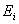=11.256 eV and the following row of excitation energies corresponding to the stationary energy levels : 7.48; 7.68; 7.95; 9.68; 9.71; 9.83; 10.38; 10.39; 10.40; 10.42; 10.43; 10.71; 10.72; 10.73; 10.88;

10.89; 10.99; 13.12 eV is considered to be the most active valency electron. The first three underlined values are so close that we have every reason to suppose that they belong to the triplet, that’s why let us find their average value (7.48+7.69+7.95)/3=7.70 eV. Then the difference of energies will be equal to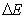=11.26-7.70=3.56 eV, and fictitious binding energy with the atomic nucleus corresponding to the first energy level will be as follows:=3.56× 4=14.24 eV. If we insert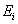=11.256 eV and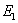=14.24 eV into the formulas (206) and (207), we’ll find (Table 20).

Table 20. Spectrum of the first electron in the    carbon  atom

 Values n 2 3 4 5 6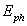(exper) eV 7.68 9.67 10.37 10.69 10.86(theor.) eV 7.7 9.68 10.38 10.71 10.88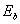(theor.) eV 3.58 1.58 0.89 0.57 0.39

Let us prepare our reader to surprises, which we can meet during the analysis of the structure of the nuclei and the atoms of chemical elements. The first surprise is in the fact that binding energy of all electrons with the atomic nuclei being in free state, which are approximately the same. On the face of it, this conflicts with the experiment, because it gives various values of binding energies of various electrons with the atomic nuclei. But one should take into consideration the conditions of the experiments, which have such differences.

The process of fixation of the spectral lines takes place under the conditions of a transition of the atoms to free state. Almost all the electrons of the atom have approximately the same opportunity to separate from it. When one electron has left the atom, the released proton in the nucleus begins to spread its influence on other electrons increasing their attraction to the nucleus and, consequently, binding energy.

Usually, the electrons from the atom are removed consequently one by one. As each of them interacts with one proton of the nucleus, the released protons begin to interact with the electrons, which remain in the atom. As a result, binding energy of the electrons remaining in the atom is increased. When one electron remains in the atom, it interacts with all protons of the nucleus, and its binding energy with the nucleus is increased proportionally to square of the number of the protons in the nucleus.

It means that all electrons of the atoms have approximately the same binding energies with the nucleus as the electron of the hydrogen atom. Later on we’ll see that this assumption is correct. Now let us calculate the spectra of two electrons of the oxygen atom.

The smallest ionization energy of the electron of the oxygen atom is equal to=13.618 eV, and binding energy of this electron with the atomic nucleus corresponding to the first energy level is equal to=13.752 eV. Let us call this electron the first one. The calculation of energy indices of this electron according to the formulae (206) and (207) give the following results (Table 21).

Table 21. Spectrum of the first electron in the oxygen atom

 Valumes n 2 3 4 5 6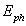(exper) eV 10.18 12.09 12.76 13.07 13.24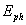(theor.) eV 10.16 12.09 12.76 13.07 13.24(theor.) eV 3.44 1.53 0.86 0.55 0.38

Ionization energy of the second electron of oxygen atom is equal to=35.116 eV, and its binding energy with the atomic nucleus corresponding to the first energy level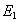=83.98 eV. We’d like to draw the attention of the readers to large divergences of experimental data concerning the second potential of ionization, which are available in the reference books  and . We trust the new data being available in the reference book . Taking into consideration the above-mentioned facts the spectrum of the second electron of oxygen atom has proved to be as follows (Table 22).

Table 22. Spectrum of the second electron of the oxygen atom

 Values n 2 3 4 5 6(exper) eV 14.12 25.83 29.81 31.73 32.88(theor.) eV 14.12 25.79 29.87 31.76 32.78(theor.) eV 21 9.33 5.25 3.36 2.33

As it is known, chlorine is the 17th element of the periodic law of chemical elements. Ionization potential of its 1st electron is=12.967 eV, and its binding energy with the atomic nucleus corresponding to the first energy level is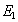=15.548 eV. Experimental and theoretical values of energiesof the photons being absorbed and emitted by this electron corresponding to various energy levels and binding energiesof this electron with the nucleus of the chlorine atom are given in Table 23.

Table 23. Spectrum of the 1st electron of the chlorine atom

 Values n 2 3 4 5 6(exper) eV 9.08 11.25 12.02 12.34 12.53(theor.) eV 9.08 11.24 11.99 12.34 12.54(theor.) eV 3.89 1.72 0.97 0.62 0.43

Copper is the 29th element of the periodic law of chemical elements. Ionization potential of its 1st electron is=7.724 eV, and its binding energy corresponding to the first energy level is=98.85 eV. The rest energy indices of this electron are given in Table 24.

Table 24.  Spectrum of the 1st electron of the copper atom

 Values n 5 6 7 8 9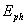(exper) eV 3.77 4.97 5.72 6.19 6.55(theor.) eV 3.77 4.98 5.71 6.18 6.5(theor.) eV 3.96 2.75 2.02 1.54 1.22

Gallium is the 31st element. Ionization potential of its 1st electron is=6.00 eV, and energy corresponding to the first energy level is=46.88 eV. Other energy indices of this electron are given in Table 25.

Table 25. Spectrum of the 31st electron of the gallium atom

 Valumes n 4 5 6 7 8(exper) eV 4.11 4.71 5.06 5.23 5.4(theor.) eV 4.12 4.7 5.04 5.27 5.42(theor.) eV 2.93 1.87 1.3 0.96 0.73

The first electron of the sodium atom (Na) has the smallest binding energies with the nucleus, that’s why it is the main valency electron of this atom (Table 26). Ionization energy of the first electron of the sodium atom is equal to=5.139 eV, and binding energy with the nucleus corresponding to the first energy level is=13.086 eV. If we insert these results into the formulas (206) and (207), we’ll find (Table 26).

Table 26. Spectrum of the 1st electron of the sodium atom

 Valumes n 2 3 4 5 6(exper) eV - 3.68 4.31 4.62 4.78(theor.) eV - 3.68 4.32 4.62 4.77(theor.) eV 3.27 1.45 0.82 0.52 0.36

In Table 26 theoretical(theor.) and experimental(exper.) values of energies of the photons emitted and absorbed by this electron are given as well as its binding energies(theor.) with the atomic nucleus calculated according to the formulas (206) and (207). We’d like to draw your attention to the fact that the second energy level of this electron is fictitious (as the first one).

Modern theories of formation of molecules establish only the value of energy being necessary, as they say, for a transfer of one electron and estimate it as being equal to 1.2-1.3 eV. This is binding energy of the electron with the nucleus of unknown energy level.

As it is clear from our analysis, each electron has a series of binding energies. A new theory gives the opportunity to calculate these energies for any energy level of the electron and to determine the number of this level and, consequently, the distance between the atomic nucleus and valency electron.

We’ll continue analysis of binding energies of the electrons with the atomic nuclei during consideration the structures of the atoms of chemical elements.

We finish the presentation of the theory of formation of the spectra of the atoms and the ions, and we should note important issues for those who are going to continue these investigations. First of all, it is only the beginning. It is based on the results of experiments. If the results of the experiment differ from the actual spectrum of this or that electron, the search process of energybecomes very complicated. As the value of this energy is based on the value of excitation energy, which is the first one in the row of all excitation energies corresponding to the stationary energy levels, the exact definition of the first excitation energy plays a decisive role. But the existing reference books concerning spectroscopy do not meet this requirement. For example, let us take excitation energies corresponding to the stationary energy levels of the second electron of the carbon atom.

The reference book written by Striganov  contains the following row of these energies: 5.33; 9.29; 11.96; 13.71; 13.72; 14.45; 18.04; 19.49; 20.84; 21.49; 22.13; 22.47; 22.57; 22.82; 23.38; 26.58 eV. In the reference book written by Zaidel, this row has the following values: 9.30; 11.96; 13.72; 14.46; 16.32; 17.62; 18.04; 18.06; 18.66; 19.49; 20.14; 20.84; 20.91; 20.95; 22.13; 22.54; 22.56; 22.90; 23.11; 24.27; 24.37; 24.59; 24.64; 25.98; 27.41; 27.47; 27.48 eV.

The underlined values of energies coincide in both reference books; the values, which are not underlined do not coincide. As it is clear, it is not easy to find energy, which corresponds to the first excitation level. It looks like that this task should be solved by means of an increase of the number of reference books used during the analysis and elaboration of a special computer program, which could provide the solution of the problem being set. If there are such rows of energies, which do not obey the law (207), it will mean that at the moment of absorption and emission of the photon, spin of the given electron is not parallel to spin of the photon. In other words: a cell of such electron occupies non-standard place in the atom.

8.9.   THE NEW INTERPRETATION OF PHOTOEFFECT

It is known that the most acceptable interpretation of experimental dependencies of photoeffect has been suggested by A. Einstein in 1905, and he got the Noble prise for it , , . He made it in the absence of the law of formation of the atom and ion spectra. Now this law has been discovered, and we can check correctness of its interpretation and the possibility of deeper understanding of photoeffect.

The mathematical model suggested by A. Einstein for the interpretation of experimental dependencies of photoeffect has the following form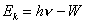,                                                                   (226)

where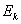is kinetic energy of the photoelectron emitted by the photocathode;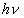is energy of the photon, but it is not explained in the papers, of which photon exactly,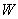is the photoelectron exit work is a constant, which does not depend on frequency . The main experimental dependencies of photeffect are given in Fig. 20 .

Photocurrent takes place in the photocathode-collector circuit. If the photocathode is exposed to monochromatic light  (Fig. 20, a), the value of potential –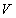retarding the photoelectrons emitted by the photocathode does not depend on light intensity. Light intensity increase increases photocurrent and does not exert influence on the retarding potential value and, consequently, on kinetic energy of the photoelectrons. If frequency of light, which strikes upon the photocathode, is increased, the value of the negative potential –retarding the photoelectrons is increased (Fig. 20, b).

As the value of the retarding negative potential –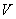is determined by kinetic energyof the electrons emitted by the photocathode under the influence of light illumination, it results from the dependence shown in Fig. 20,b that kinetic energy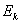of the photoelectrons emitted by them is increased with the increase of frequency of the photons exposing the photocathode.

Let us try to find a connection of Einstein’s equation (226) with the mathematical model of the law of formation of the atom and ion spectra (207).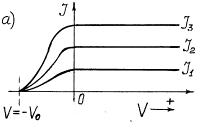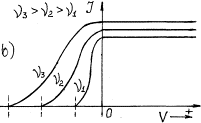Fig. 20. Dependence of photocurrent on light intensity: a) at its constant frequency; b) at different frequency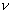The correlation (207) appears from experimental spectroscopy, that’s why it is a mathematical model of the law of formation of the atom and ion spectra. Einstein’s equation (226) describes similar process of photon absorption by the electrons. It affords ground for supposition of identity of  the equations (207) and (226) and uniformity of their interpretation. It appears from the given equations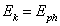.                                                                     (227)

It means that if the electron loses bond with the atomic nucleus, its kinetic energyis equal to energyof the absorbed photon. Then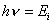.                                                                           (228)

It appears from this thatenergy value in Einstein’s equation (226) is ionization energyof the electron emitted by the photocathode material. It appears from the equations (207) and (226) that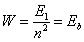.                                                                         (229)

The new clarification: photoelectron exit workis equal to binding energy of electron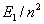when it is on a definite energy level in the atom or the molecule.

Experimental investigations of photo effect are carried out usually with the photocathodes made of alkali metals . For example, it is known that the work of the photoelectron exit from the lithium photocathode is equal to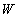=2.4 eV . Ionization energy of this electron is equal to=5.392 eV, and its binding energy with the nucleus corresponding to the first energy level is equal to=14.05 eV. If we take it into account and use the mathematical model of the law of formation of the atom and ion spectra (207), we’ll get a theoretical spectrum of this electron(theor.), which coincides completely with the experimental spectrum(exp.) (Table 15). Formula (229) gives an opportunity to calculate binding energiesof this electron with the atomic nucleus  (according to Einstein, the exit work), which correspond to all (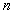) energy levels of this electron (Table 15).

It is known that the like atoms are united in a molecule with the covalent bond, and binding energies between valence electrons correspond to fractional quantum numbers. It allows us to determine what is a photoelectron emission source: atoms or molecules of the photocathode material. If we substitute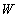=2.4 eV and=14.05 eV in the formula (229), we’ll find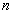=2.4. As valueis a fractional number, it means that the lithium molecules, not atoms are the source of the photoelectrons (Table 15).

For a photoelectron of the sodium photocathode, we have:=5.139 eV,=13.086 eV and=2.1 eV [24.] If we use the mathematical model of the law of formation of the atom and ion spectra (207), we’ll get a sodium photoelectron spectrum (Table 26).

Valuedetermined with the help of the formula (229) is equal to=2.5. It appears from this that the sodium molecules, not atoms are the source of the photoelectrons of the sodium photocathode.

The mathematical model of the law of formation of the atom and ion spectra (207) shows that it has no orbital component of electron energy. It appears from this that the electron has no orbital motion in the atom. The molecules are formed by means of a combination of the unlike magnetic poles of their valence atomic electrons, which are connected with the nuclear protons by means of the magnetic poles as well.

The analysis of the law of formation of the atom and ion spectra (207) as well as the spectrum calculation results (Table 15 and 26) show that binding energyof the electron with the atomic nucleus and, consequently, binding energy of valence electrons of two atoms with each other is changed step by step (229). It appears from this that kinetic energy of the photoelectronsand value of retarding potential –(Fig. 20, b) should be changed step by step as well. The photoelectrons can absorb only the photons, which correspond to their binding energies in the molecules of the given substance. The greater binding energy between the electrons in the molecules, the greater photon energy is required for the break of this bond and the greater kinetic energy the photoelectrons being released will acquire, and the greater potential will be required for their retardation on the way to the collector. Let us pay attention to the fact that the given logical chain originates from the mathematical model of the law of formation of the atom and ion spectra (207) and is present in Einstein’s equation implicitly (226).

Current is present in the circuit due to the fact that the photoelectrons emitted by the photocathode material molecules are substituted by free electrons. They should emit the photons, which energy is equal to binding energy of the electrons in the molecules, but light striking the photocathode does not allow us to see this emission.

Mathematical Einstein’s equation (226) describing experimental regularities of photo effect has deeper physical sense. When the components of this equation are interpreted correctly, it becomes the mathematical model  (207) of the law of formation of the atom and ion spectra discovered by us in 1993 and published in the papers , , , .

The Foundations of Physchemistry of Microworld

Ó2003 Kanarev Ph. M.

Internet Version - http://book.physchemistry.innoplaza.net

<< Back to Physchemistry Book Index